# PyTorch 12：nn 网络层：卷积层

## PyTorch 学习笔记

Posted by YEY on December 16, 2020

# Lecture 12 nn 网络层：卷积层

## 1. 一维、二维和三维卷积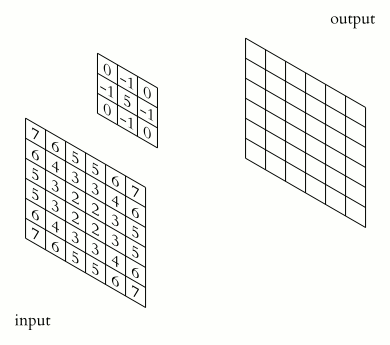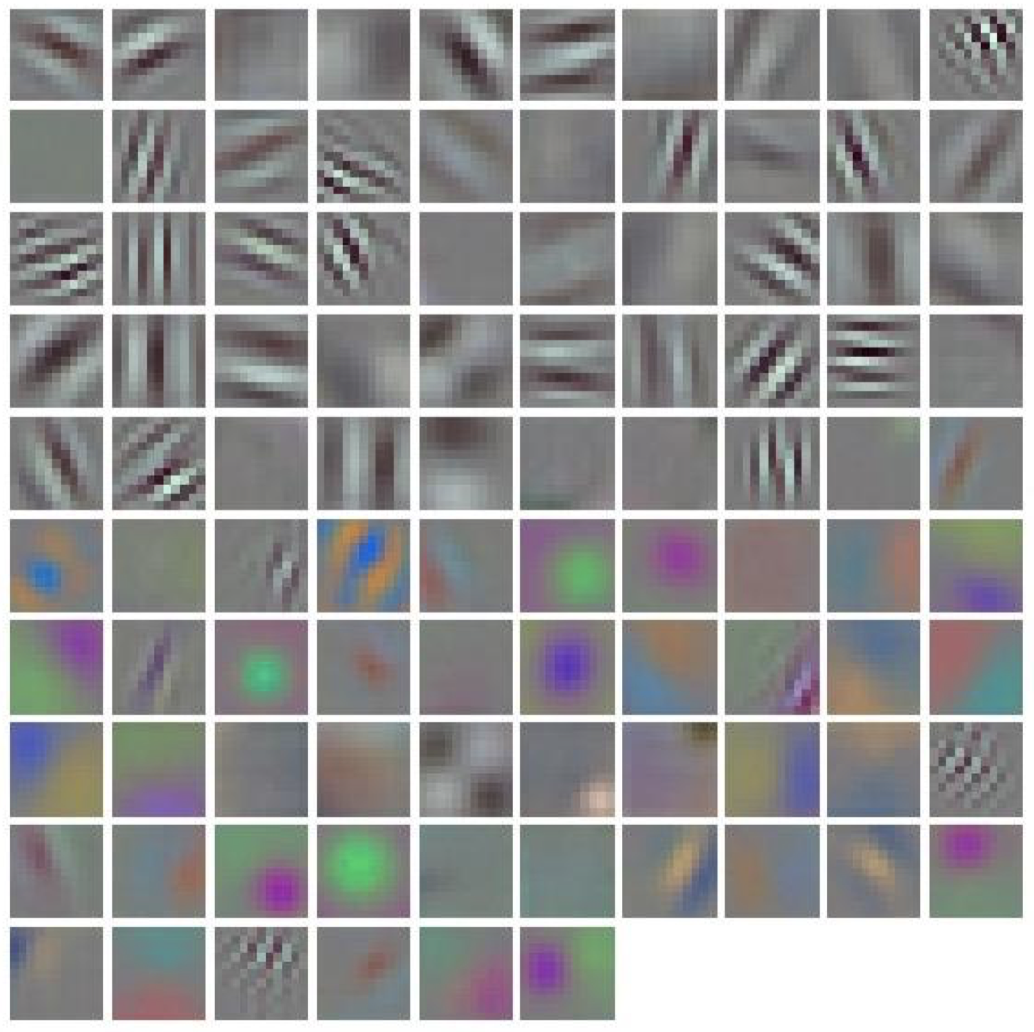1d 卷积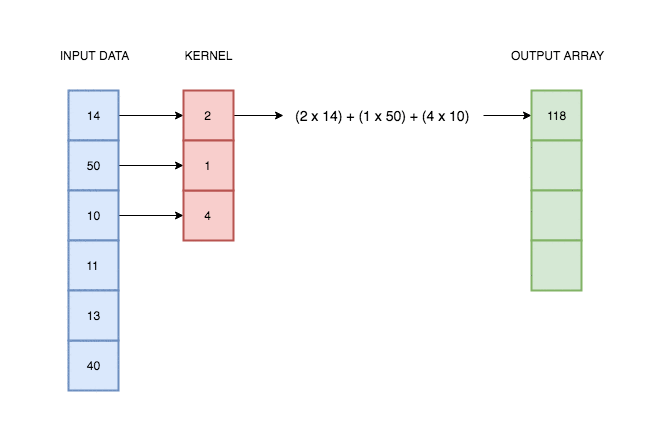2d 卷积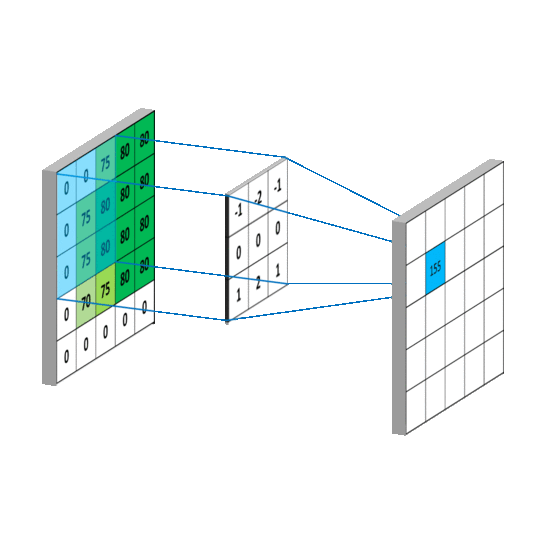3d 卷积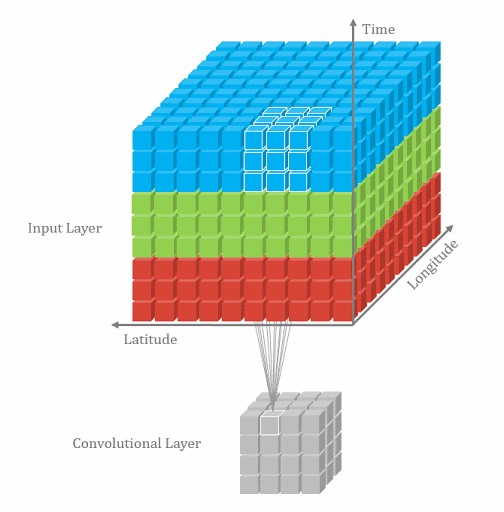## 2. 二维卷积

#### nn.Conv2d

1
2
3
4
5
6
7
8
9
10
11
nn.Conv2d(
in_channels,
out_channels,
kernel_size,
stride=1,
dilation=1,
groups=1,
bias=True,
)


• in_channels：输入通道数。
• out_channels：输出通道数，等价于卷积核个数。
• kernel_size：卷积核尺寸。
• stride：步长。下面是一个步长为 2 的卷积：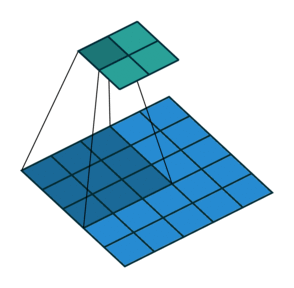• padding：填充个数。常用于保持输入输出图像尺寸匹配，可以用于提高输出图像的分辨率：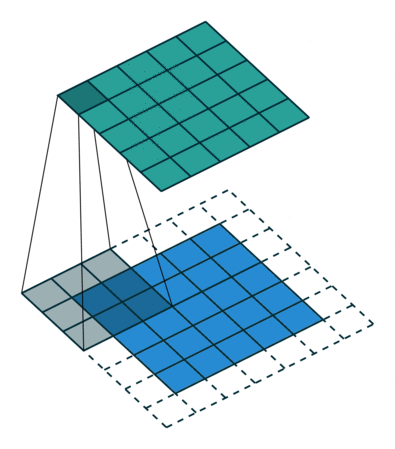• dilation：空洞卷积大小。常用于图像分割任务，目的是提高感受野，即输出图像的一个像素对应输入图像上更大的一块区域：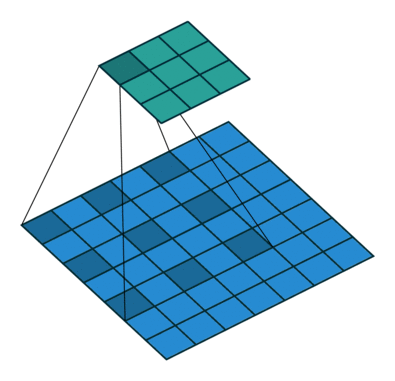• groups：分组卷积的组数。常用于模型的轻量化。例如，Alexnet 当时由于硬件限制采用了两组卷积操作：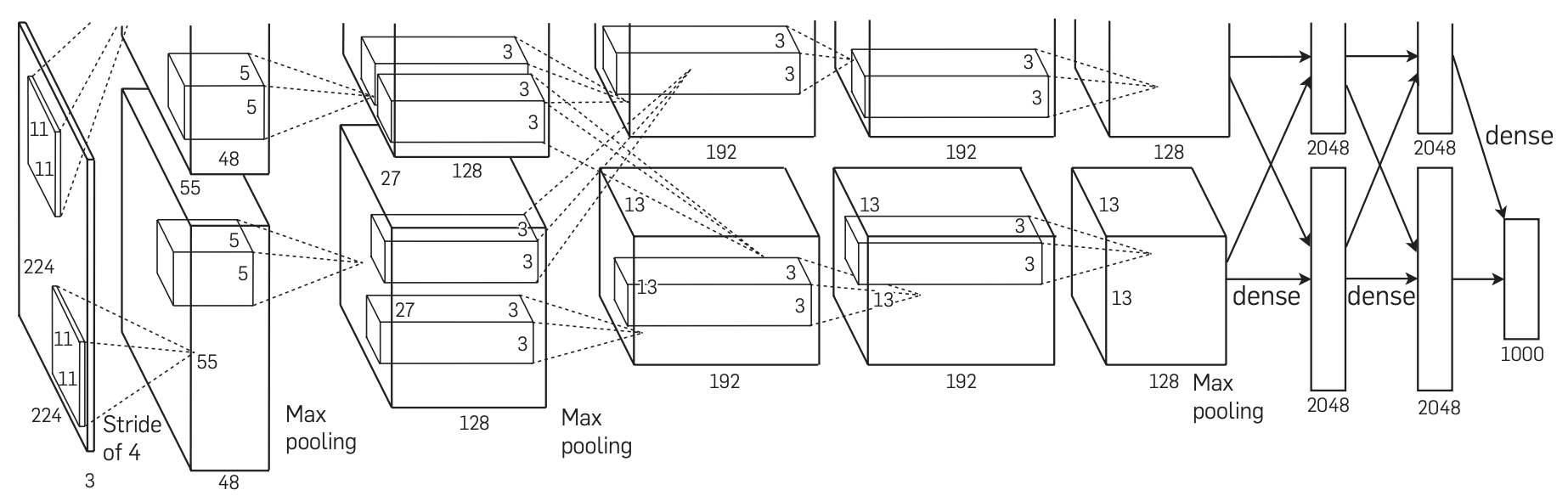• bias：偏置。最终输出响应值时需加上偏置项。

• 简化版 (不带 paddingdilation)：

$\mathrm{out}_{\text{size}} = \dfrac{\mathrm{in}_{\text{size}} - \mathrm{kernel}_{\text{size}}}{\mathrm{stride}} + 1$
• 完整版：

$H_{\text{out}} = \left\lfloor \dfrac{H_{\text{in}} + 2\times \mathrm{padding} - \mathrm{dilation} \times (\text{kernel_size}-1)-1}{\mathrm{stride}} + 1 \right\rfloor$

1
2
3
4
5
6
7
8
9
10
11
12
13
14
15
16
17
18
19
20
21
22
23
24
25
26
27
28
29
30
31
32
import os
import torch.nn as nn
from PIL import Image
from torchvision import transforms
from matplotlib import pyplot as plt
from tools.common_tools import transform_invert, set_seed

set_seed(3)  # 设置随机种子，用于调整卷积核权值的状态。

path_img = os.path.join(os.path.dirname(os.path.abspath(__file__)), "lena.png")
img = Image.open(path_img).convert('RGB')  # 0~255

# convert to tensor
img_transform = transforms.Compose([transforms.ToTensor()])
img_tensor = img_transform(img)
img_tensor.unsqueeze_(dim=0)    # C*H*W to B*C*H*W

# ========================= create convolution layer ==========================
conv_layer = nn.Conv2d(3, 1, 3)   # input:(i, o, size) weights:(o, i , h, w)
nn.init.xavier_normal_(conv_layer.weight.data)

# calculation
img_conv = conv_layer(img_tensor)

# =========================== visualization ==================================
print("卷积前尺寸:{}\n卷积后尺寸:{}".format(img_tensor.shape, img_conv.shape))
img_conv = transform_invert(img_conv[0, 0:1, ...], img_transform)
img_raw = transform_invert(img_tensor.squeeze(), img_transform)
plt.subplot(122).imshow(img_conv, cmap='gray')
plt.subplot(121).imshow(img_raw)
plt.show()


1
2


• set.seed(1) 时的输出：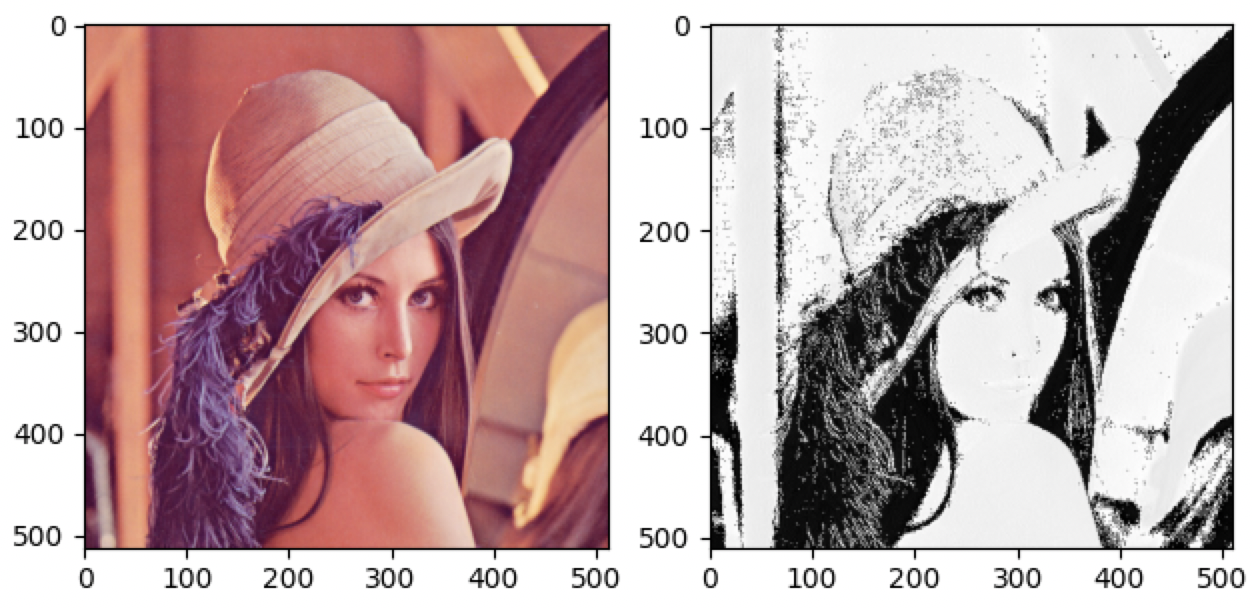• set.seed(2) 时的输出：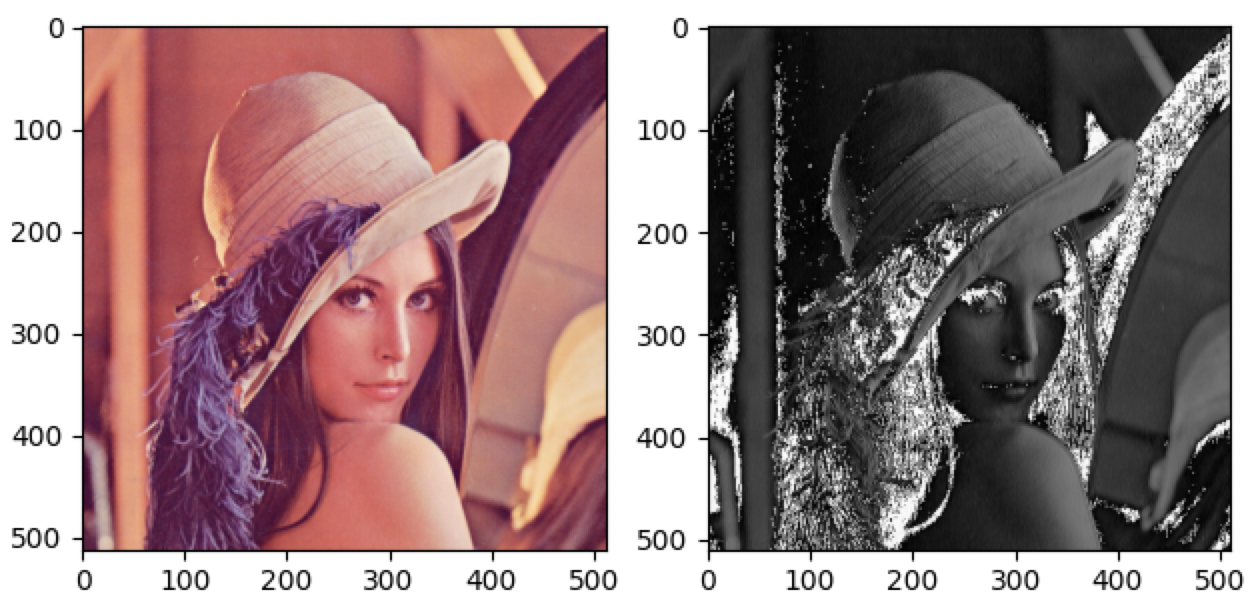• set.seed(3) 时的输出：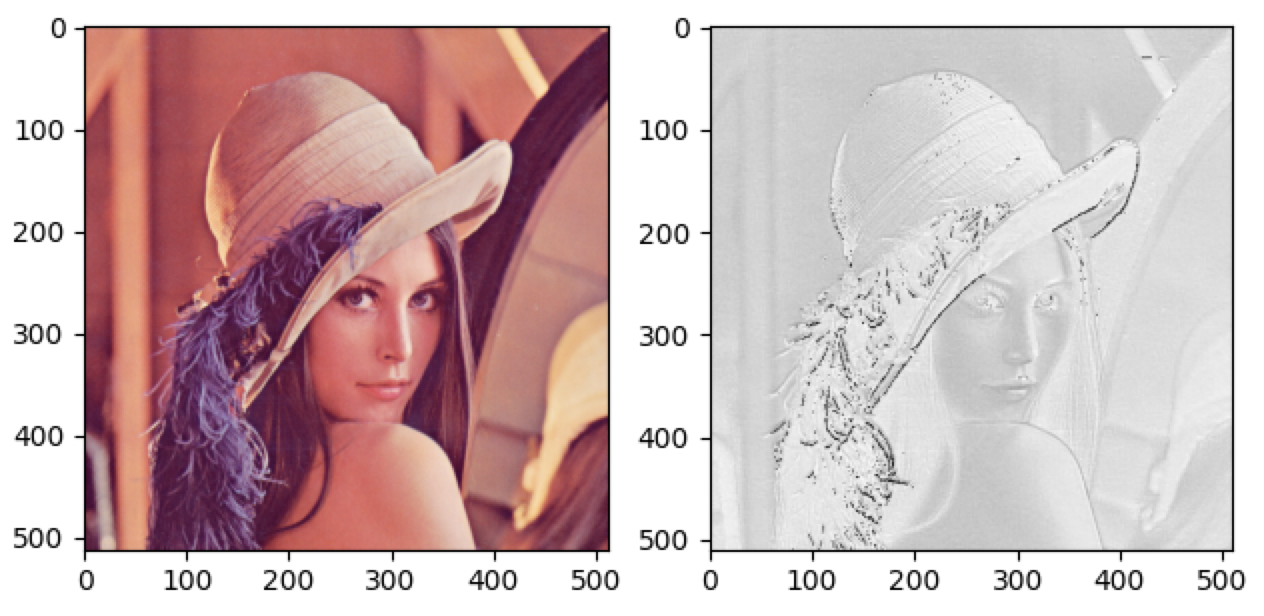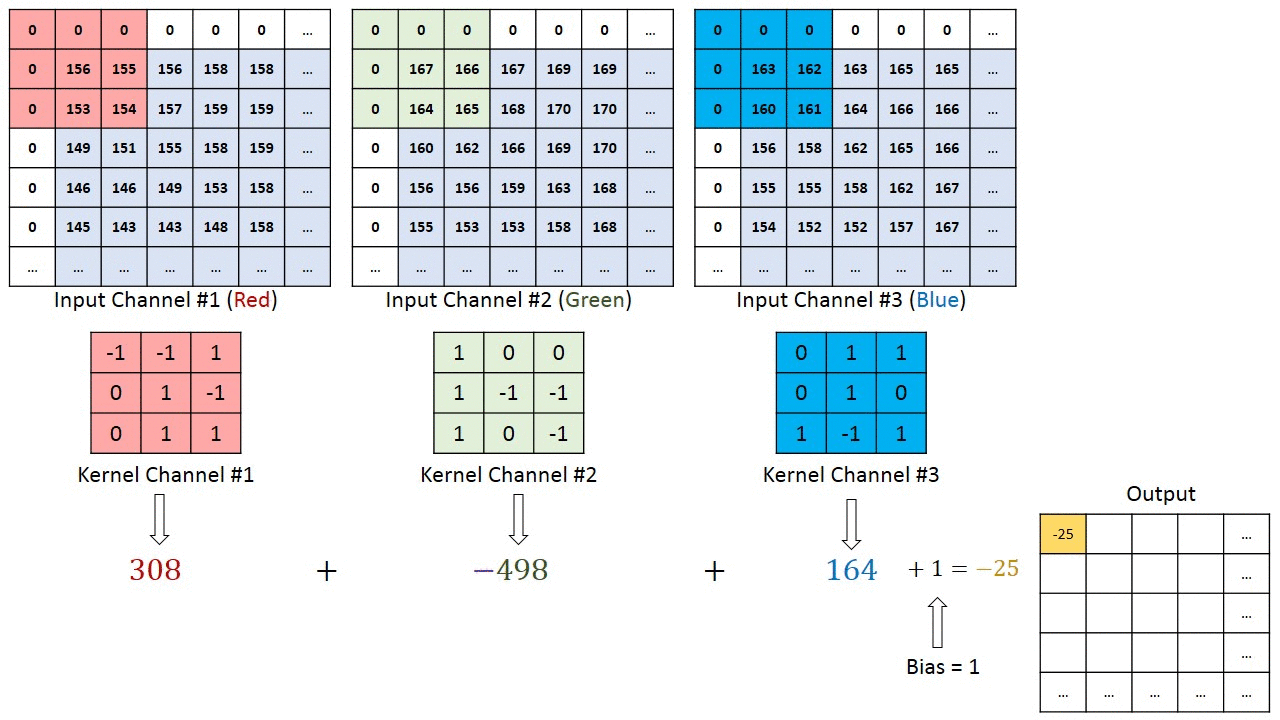## 3. 转置卷积

(注 1：这里我们说的反卷积不同于信号系统中的反卷积)。

• 正常卷积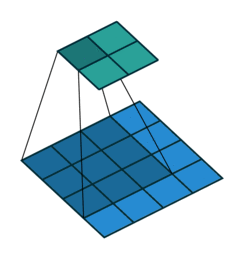假设图像尺寸为 $4 \times 4$，卷积核为 $3\times 3$，$\mathrm{padding} = 0$，$\mathrm{stride} = 1$。

• 图像：$I_{16\times 1}$，这里 $16$ 是输入图像的像素总数，$1$ 表示图片张数。
• 卷积核：$K_{4\times 16}$，这里 $4$ 是输出图像的像素总数，$16$ 是由卷积核中的 $9$ 个元素另外补零后得到。
• 输出：$O_{4\times 1} = K_{\color{orange}{4\times 16}} \times I_{16\times 1}$

• 转置卷积：上采样，输出图像比输入图像尺寸更大。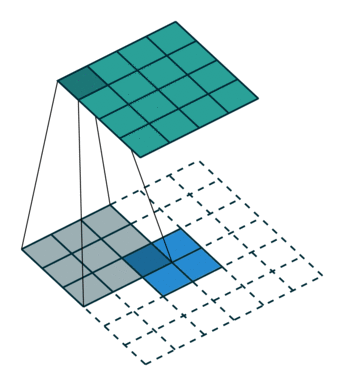假设图像尺寸为 $2 \times 2$，卷积核为 $3\times 3$，$\mathrm{padding} = 0$，$\mathrm{stride} = 1$。

• 图像：$I_{4\times 1}$，这里 $4$ 是输入图像的像素总数，$1$ 表示图片张数。
• 卷积核：$K_{16\times 4}$，这里 $16$ 是输出图像的像素总数，$4$ 是由卷积核中的 $9$ 个元素剔除一部分后得到。
• 输出：$O_{16\times 1} = K_{\color{orange}{16\times 4}} \times I_{4\times 1}$

#### nn.ConvTranspose2d

1
2
3
4
5
6
7
8
9
10
11
12
nn.ConvTranspose2d(
in_channels,
out_channels,
kernel_size,
stride=1,
groups=1,
bias=True,
dilation=1,
)


• in_channels：输入通道数。
• out_channels：输出通道数。
• kernel_size：卷积核尺寸。
• stride：步长。
• padding：填充个数。
• dilation：空洞卷积大小。
• groups：分组卷积设置。
• bias：偏置。

• 简化版 (不带 paddingdilation)：

$\mathrm{out}_{\text{size}} = (\mathrm{in}_{\text{size}} -1)\times \mathrm{stride} + \mathrm{kernel}_{\text{size}}$
• 完整版：

$H_{\text{out}} = (H_{\text{in}}-1) \times \mathrm{stride} - 2 \times \mathrm{padding} + \mathrm{dilation} \times (\text{kernel_size}-1) + \mathrm{output\_padding}+ 1$

1
2
3
4
5
6
7
8
9
10
11
12
13
14
15
16
17
18
19
20
21
22
23
24
25
26
27
28
29
30
31
32
import os
import torch.nn as nn
from PIL import Image
from torchvision import transforms
from matplotlib import pyplot as plt
from tools.common_tools import transform_invert, set_seed

set_seed(3)  # 设置随机种子，用于调整卷积核权值的状态。

path_img = os.path.join(os.path.dirname(os.path.abspath(__file__)), "lena.png")
img = Image.open(path_img).convert('RGB')  # 0~255

# convert to tensor
img_transform = transforms.Compose([transforms.ToTensor()])
img_tensor = img_transform(img)
img_tensor.unsqueeze_(dim=0)    # C*H*W to B*C*H*W

# ========================= create convolution layer ==========================
conv_layer = nn.ConvTranspose2d(3, 1, 3, stride=2)   # input:(i, o, size)
nn.init.xavier_normal_(conv_layer.weight.data)

# calculation
img_conv = conv_layer(img_tensor)

# =========================== visualization ==================================
print("卷积前尺寸:{}\n卷积后尺寸:{}".format(img_tensor.shape, img_conv.shape))
img_conv = transform_invert(img_conv[0, 0:1, ...], img_transform)
img_raw = transform_invert(img_tensor.squeeze(), img_transform)
plt.subplot(122).imshow(img_conv, cmap='gray')
plt.subplot(121).imshow(img_raw)
plt.show()


1
2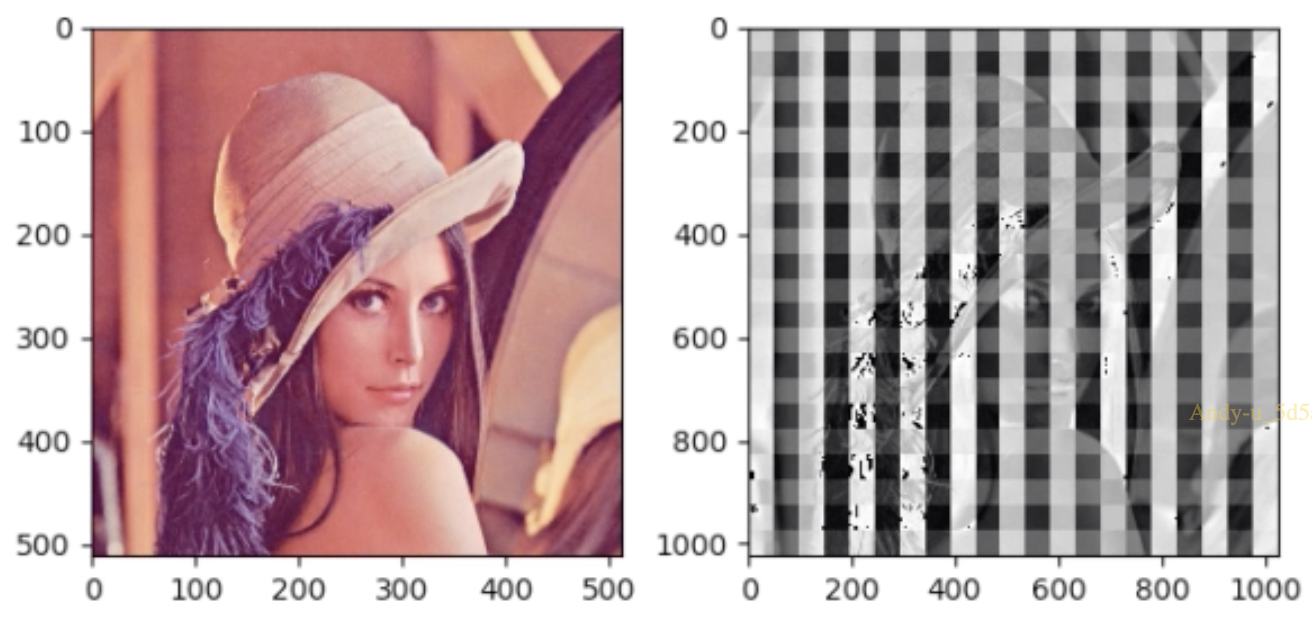## 4. 总结本作品采用知识共享署名-非商业性使用-相同方式共享 4.0 国际许可协议进行许可。 欢迎转载，并请注明来自：YEY 的博客 同时保持文章内容的完整和以上声明信息！